# 动态规划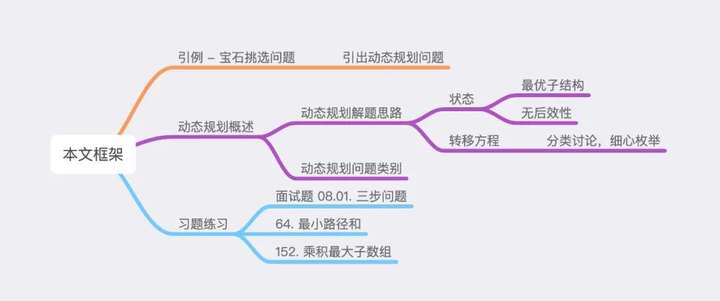## 一、宝石挑选

### ​问题引入

​小 Q 可以免费带走一个连续区间中的宝石，比如区间或区间中的宝石。

### 问题分析显然有些无法接受，因此想想有没有办法优化，比如优化掉暴力累加的部分。

### 优化 2.0​在上述推导过程中，一共分为两步：

1. 将整个问题划分为一个个子问题，并令为第个子问题的答案

2. 思考大规模的子问题如何从小规模的子问题推导而来，即如何由推出## 二、动态规划概述

### DP 状态

「DP 状态」的确定主要有两大原则：

1. 最优子结构
2. 无后效性

### DP 转移方程### 动态规划问题类别

DP 问题主要分为两大类，第一大类是 DP 类型，第二大类是 DP 优化方法。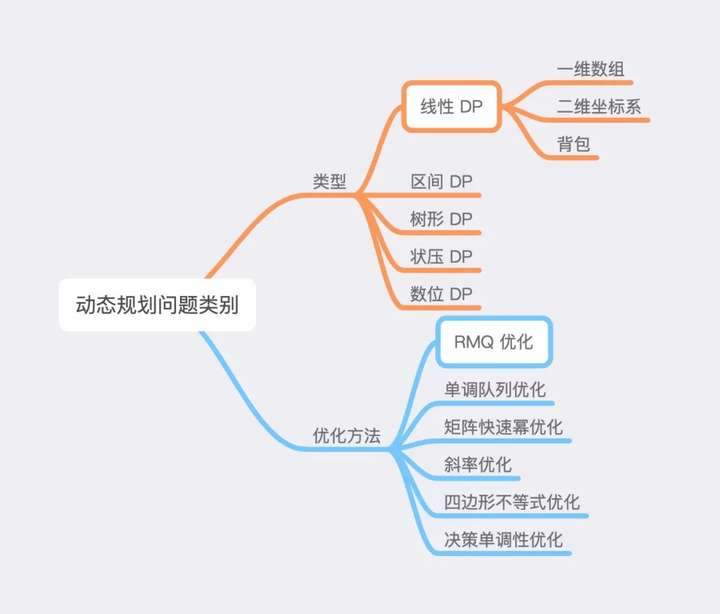## 三、习题练习

### 题目描述

输入：n = 3



输入：n = 5



n 范围在 [1, 1000000] 之间


### 解题思路

DP 问题思路主要就是确定「DP 状态」与「DP 转移方程」，因此我们首先考虑「DP 状态」。

「DP 状态」的确定有两大原则，一是「最优子结构」，二是「无后效性」，简要概括就是将原问题划分为多个子问题，且「大规模子问题最优值」仅与「小规模子问题最优值」有关，与「小规模子问题最优值」是如何得到的无关。表示爬阶楼梯的总方案数，原问题被划分为了多个求最优值的子问题，继续思考，不难发现小孩爬楼梯只有三种选项，一次上 1、2、3 阶，因此的值仅由的值决定，因此符合「最优子结构」原则。​C++ 代码实现

class Solution {
public:
vector<int> f;
int mod = 1000000007;
int waysToStep(int n) {
f.resize(n+1);
f = 1;
for(int i = 1; i <= n; i++) {
f[i] = f[i-1];
if(i >= 2) f[i] = (f[i] + f[i-2]) % mod;
if(i >= 3) f[i] = (f[i] + f[i-3]) % mod;
}
return f[n];
}
};



## 64. 最小路径和

### 题目描述

**说明：**每次只能向下或者向右移动一步。

### 示例 1:

输入:
[
[1,3,1],
[1,5,1],
[4,2,1]
]



### 解题思路表示从左上角到坐标的路径数字和最小值，原问题即可被划分为多个求最优值的子问题，且由于每次只能向下或向右移动一步，因此的取值由的值决定，即符合「最优子结构原则」。C++ 代码实现

class Solution {
public:
int minPathSum(vector<vector<int>>& grid) {
for(int i = 0; i < grid.size(); i++)
for(int j = 0; j < grid.size(); j++) {
if(i == 0 && j == 0) continue;
int tp = 1e9;
if(i > 0) tp = min(tp, grid[i-1][j]);
if(j > 0) tp = min(tp, grid[i][j-1]);
grid[i][j] += tp;
}
return grid[grid.size()-1][grid.size()-1];
}
};



## 152. 乘积最大子数组

### 示例 1:

输入: [2,3,-2,4]



输入: [-2,0,-1]



### 解题思路

••「以为右端点的连续区间最小乘积」*if(nums[i] > 0) {
maxn[i] = max(nums[i], maxn[i - 1] * nums[i]);
minn[i] = min(nums[i], minn[i - 1] * nums[i]);
}
else {
maxn[i] = max(nums[i], minn[i - 1] * nums[i]);
minn[i] = min(nums[i], maxn[i - 1] * nums[i]);
}



### C++ 代码实现

class Solution {
public:
vector<int> maxn, minn;
int maxProduct(vector<int>& nums) {
int n = nums.size(), ans = nums;
maxn.resize(n);
minn.resize(n);
maxn = minn = nums;
for (int i = 1; i < nums.size(); ++i) {
if(nums[i] > 0) {
maxn[i] = max(nums[i], maxn[i - 1] * nums[i]);
minn[i] = min(nums[i], minn[i - 1] * nums[i]);
}
else {
maxn[i] = max(nums[i], minn[i - 1] * nums[i]);
minn[i] = min(nums[i], maxn[i - 1] * nums[i]);
}
ans = max(ans, maxn[i]);
}
return ans;
}
};



## 总结

• 确定「DP 状态」

• 符合「最优子结构」原则：DP 状态最优值由更小规模的 DP 状态最优值推出

• 符合「无后效性」原则：状态的得到方式，不会影响后续其它 DP 状态取值

• 确定「DP 转移方程」

• 分类讨论，细心枚举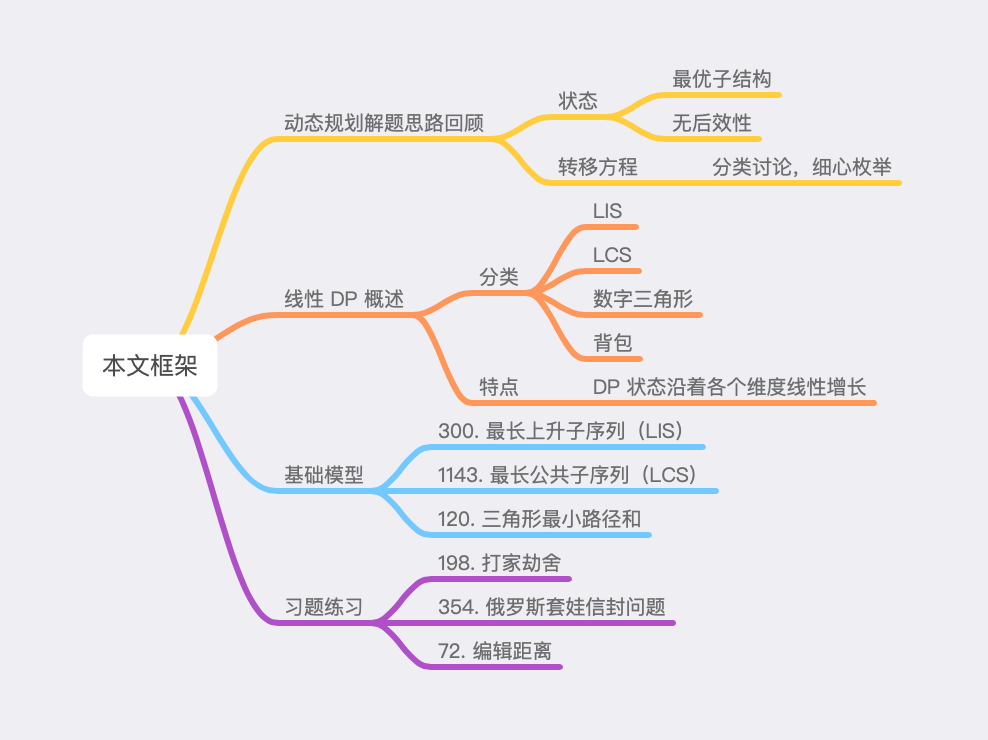## 一、动态规划解题思路回顾

「DP 状态」的确定有两大原则，一是「最优子结构」，二是「无后效性」，简要概括就是将原问题划分为多个子问题，且「大规模子问题最优值」仅与「小规模子问题最优值」有关，与「小规模子问题最优值」是如何得到的无关。

## 二、线性 DP 概述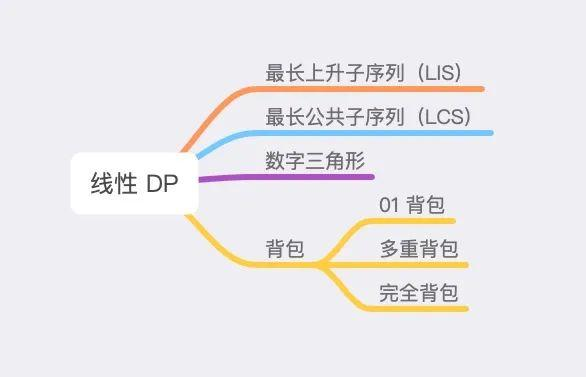## 三、基础模型

### 300. 最长上升子序列（LIS）

a​leetcode-cn.com

### 示例​

输入: [10,9,2,5,3,7,101,18]



### 模型讲解C++ 代码实现

class Solution {
public:
int lengthOfLIS(vector<int>& nums) {
int sz = nums.size(), ans = 0;
vector<int> f(sz, 0);
for(int i = 0; i < sz; i++) {
int tmp = 1;
for(int j = i-1; j >= 0; j--) {
if(nums[i] > nums[j])
tmp = max(tmp, f[j]+1);
}
f[i] = tmp;
ans = max(ans, tmp);
}
return ans;
}
};



1143. 最长公共子序列（LCS）

a​leetcode-cn.com

### 题目描述

输入：text1 = "abcde", text2 = "ace"



输入：text1 = "abc", text2 = "abc"



输入：text1 = "abc", text2 = "def"



••• 输入的字符串只含有小写英文字符。LCS 作为最基本的双串匹配 DP 模型，其转移方式考察较为频繁，大家需要好好把握理解。该算法时间复杂度为分别为串的长度。

C++ 代码实现

class Solution {
public:
int longestCommonSubsequence(string text1, string text2) {
int n = text1.length(), m = text2.length();
vector<vector<int> > f(n+1, vector<int>(m+1, 0));
for(int i = 1; i <= n; i++) {
for(int j = 1; j <= m; j++) {
f[i][j] = max(f[i-1][j], f[i][j-1]);
if(text1[i-1] == text2[j-1])
f[i][j] = max(f[i][j], f[i-1][j-1]+1);
}
}
return f[n][m];
}
};



## 120. 三角形最小路径和

a​leetcode-cn.com

### 题目描述

[
,
[3,4],
[6,5,7],
[4,1,8,3]
]


### 模型讲解### C++ 代码实现

class Solution {
public:
int minimumTotal(vector<vector<int>>& triangle) {
int n = triangle.size(), ans = 1e9;
vector<vector<int> > f(n+1, vector<int>(n+1, 0));
for(int i = 0; i < n; i++) {
for(int j = 0; j < triangle[i].size(); j++) {
if(j == triangle[i].size()-1)
f[i+1][j+1] = triangle[i][j] + f[i][j];
else if(j == 0)
f[i+1][j+1] = triangle[i][j] + f[i][j+1];
else
f[i+1][j+1] = triangle[i][j] + min(f[i][j+1], f[i][j]);
if(i == n-1)
ans = min(ans, f[i+1][j+1]);
}
}
return ans;
}
};



### 滚动数组优化for(int i = 0; i < n; i++) {
for(int j = triangle[i].size()-1; j >= 0; j--) {
f[j] = triangle[i][j] + min(f[j-1], f[j]);
}
}



class Solution {
public:
int minimumTotal(vector<vector<int>>& triangle) {
int n = triangle.size(), ans = 1e9;
vector<int> f(n+1, 0);
for(int i = 0; i < n; i++) {
for(int j = triangle[i].size()-1; j >= 0; j--) {
if(j == triangle[i].size()-1)
f[j+1] = triangle[i][j] + f[j];
else if(j == 0)
f[j+1] = triangle[i][j] + f[j+1];
else
f[j+1] = triangle[i][j] + min(f[j+1], f[j]);
if(i == n-1)
ans = min(ans, f[j+1]);
}
}
return ans;
}
};



## 四、习题练习

1. 如何识别这是一道「线性 DP」问题
2. 「DP 状态」是如何设置的
3. 如何根据「DP 状态」得到「DP 转移方程」

### 198. 打家劫舍

a​leetcode-cn.com

### 题目描述

输入：[1,2,3,1]

偷窃到的最高金额 = 1 + 3 = 4 。


输入：[2,7,9,3,1]

偷窃到的最高金额 = 2 + 9 + 1 = 12 。


••### 解题思路C++ 代码实现

class Solution {
public:
int rob(vector<int>& nums) {
int n = nums.size();
if(n == 0) return 0;
vector<int> f(n, 0);
for(int i = 0; i < n; i++) {
f[i] = nums[i];
if(i >= 2) f[i] = max(f[i], f[i-2]+nums[i]);
if(i >= 1) f[i] = max(f[i], f[i-1]);
}
return f[n-1];
}
};



## 354. 俄罗斯套娃信封问题

a​leetcode-cn.com

### 示例

输入: envelopes = [[5,4],[6,4],[6,7],[2,3]]



### 解题思路### C++ 代码实现

class Solution {
public:
int maxEnvelopes(vector<vector<int>>& envelopes) {
sort(envelopes.begin(), envelopes.end());
int n = envelopes.size(), ans = 0;
vector<int> f(n, 0);
for(int i = 0; i < n; i++) {
int tmp = 0;
for(int j = 0; j < i; j++) {
if(envelopes[j] < envelopes[i] && envelopes[j] < envelopes[i])
tmp = max(tmp, f[j]);
}
f[i] = tmp + 1;
ans = max(f[i], ans);
}
return ans;
}
};



## 72. 编辑距离

a​leetcode-cn.com

### 题目描述

1. 插入一个字符
2. 删除一个字符
3. 替换一个字符

输入：word1 = "horse", word2 = "ros"

horse -> rorse (将 'h' 替换为 'r')
rorse -> rose (删除 'r')
rose -> ros (删除 'e')


输入：word1 = "intention", word2 = "execution"

intention -> inention (删除 't')
inention -> enention (将 'i' 替换为 'e')
enention -> exention (将 'n' 替换为 'x')
exention -> exection (将 'n' 替换为 'c')
exection -> execution (插入 'u')


### 解题思路C++ 代码实现

class Solution {
public:
int minDistance(string word1, string word2) {
int n = word1.length(), m = word2.length();
vector<vector<int> > f(n+1, vector<int>(m+1, 0));
for(int i = 1; i <= n; i++) f[i] = i;
for(int j = 1; j <= m; j++) f[j] = j;
for(int i = 1; i <= n; i++) {
for(int j = 1; j <= m; j++) {
if(word1[i-1] == word2[j-1]) f[i][j] = f[i-1][j-1];
else f[i][j] = min(f[i-1][j-1]+1, min(f[i][j-1]+1, f[i-1][j]+1));
}
}
return f[n][m];
}
};



## 总结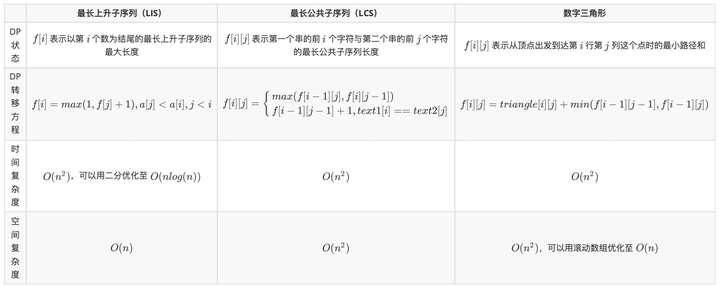# 算法